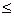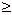edHelper subscribers - Create a new printable

Sample edHelper.com - LinearEquations Worksheet

 Name _____________________________Date ___________________
Linear Equations
Graph each system of equations and state its solution.
1.
y =
 12
x   -
 152

2x + y = 5

2.   5x - 4y = -16
y =
 54
x   +  4

Graph each system of equations and state its solution.
3.   x - 3y = -8
 2 = 2 y + -4 x

4.
 y = 2 x + 5

4y = -1 + x

Solve each system of equations using the substitution method.
 5 x + 3y = -863x + y = 38
 6 16x + 9y = 195x + 8y = -25

Solve each system of equations using the substitution method.
7.
x =
 74
y  -
 632

18x - y = -628

 8 2x + 3y = -20x + 3y = -55

Solve each system of equations using the elimination method.
 9 16x - 11y = -3118x + 5y = 469
 10 17x + 14y = -107915x + 14y = -1013

Solve each system of equations using the elimination method.
11.   5y = -112 + 3x
 1423
+
 113
y  = x

12.   -2x = 36 + 4y
y =
 -89
x   -
 623

Solve each system of equations using any method.
 13 7x + 9y = -14515x + 7y = 107
 14 8x - 5y = -222x - y = -10

Solve each system of equations using any method.
15.
y =
 -12
x   +
 932

2x + y = 72

16.
y =
 43
x   +
 473

18x + 17y = -181

Solve each system by graphing. Find the vertices of the graph.
 17 4x - 3y37x - 8y-273x + 5y35
 18 5x - y-53y-305x + 2y-20
 19 7x - 2y422x - 3y-55x + y30

Solve each system by graphing. Find the vertices of the graph.
 20 9x - 4y-203x - y-154x - 3y-154x + y-20
 21 x + y-7x + 2y116x - 11y4313x - y35
 22 2x - 3y-213x - 2y-411x - 2y154x + y2

Solve each system by graphing. Find the vertices of the graph.
 23 4x - 5y-122x - y-613x - 8y2717x - 4y3
 24 8x - 3y463x - 2y1911x - 5y58
 25 4x - y279x - 8y555x - 2y115y-15

Find the minimum and maximum values of the objective quantity.
 26 x + y511x - 5y79x - 7y-35f(x,y) = -8x + 11y - 16
 27 10x - 3y253x - 4y-84x + 5y-21f(x,y) = -4x - 3y
 28 5x - 13y333x + 5y7x - y13f(x,y) = 12x - 8y

Find the minimum and maximum values of the objective quantity.
 29 13x - 5y87x - y63x - 2y159x - 4y64f(x,y) = -9x - 9y
 30 3x + 2y-40x + y-63x - 7y324x - 5y-15f(x,y) = -7x - 12y + 8
 31 4x - 7y645x - y182x - 5y385x - 4y27f(x,y) = -11x - 10y

Find the minimum and maximum values of the objective quantity.
 32 3x - 2y144y-4x - y12x - 3y6f(x,y) = -11x - 8y
 33 4x - 5y135y-454x-12f(x,y) = -3x - 10y
 34 x + 7y-3x + y-32x - y9f(x,y) = 6x - 3y

Solve each system of equations.
 35 2x + 2y + 2z = -16-2x = 10-x + 5y + 2z = -10
 36 -2x + y - 2z = -64x = -24-x - 4y - z = 15
 37 -8x = 642x + 5y - z = 3x - 12y - 6z = 22

Solve each system of equations.
 38 -2x - 9y + 4z = -60-7x + 11y + 10z = 45-12x - y - 6z = -42
 39 -8x + 12y - 4z = 68-2x + 11y + 7z = -71-9x - 8y + 5z = 34
 40 x - 10y + 3z = -78-4x - 4y - z = 43x - 6y + 6z = -90

Sample
This is only a sample worksheet.

edHelper subscribers - Create a new printable